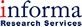# Best TX HELOC RatesTexas Home Equity Line of Credit Rates

## Compare home equity line of credit (HELOC) rates in Texas

(Last Updated: 11/13/2019)
Home Equity Lenders
APR
Rate
Initial Draw
Contact details
APR Rate \$10,000 Min. Initial Draw Amount
Conditions:
\$50,000 min.
• No Application or Appraisal Fees
• Apply Online Now
• Fixed rate installment loan
APR Rate \$0 Min. Initial Draw Amount
Intro APR
After Intro PeriodIntro Period: 12 months
Rate \$0 Min. Initial Draw Amount
Intro APR
After Intro PeriodIntro Period: 6 months
Rate \$0 Min. Initial Draw Amount
APR Rate \$50,000 Min. Initial Draw Amount
APR Rate \$0 Min. Initial Draw Amount
Intro APR
After Intro PeriodIntro Period: 4 months
Rate \$0 Min. Initial Draw Amount
Intro APR
After Intro PeriodIntro Period: 6 months
Rate \$0 Min. Initial Draw Amount
APR Rate \$0 Min. Initial Draw Amount
APR Rate \$0 Min. Initial Draw Amount
APR Rate \$50,000 Min. Initial Draw Amount
Intro APR
After Intro PeriodIntro Period: 12 months
Rate \$0 Min. Initial Draw Amount
Intro APR
After Intro PeriodIntro Period: 12 months
Rate \$0 Min. Initial Draw Amount
APR Rate \$0 Min. Initial Draw Amount
APR Rate \$0 Min. Initial Draw Amount
Intro APR
After Intro PeriodIntro Period: 12 months
Rate \$0 Min. Initial Draw Amount
Intro APR
After Intro PeriodIntro Period: 12 months
Rate \$15,000 Min. Initial Draw Amount
Intro APR
After Intro PeriodIntro Period: 6 months
Rate \$0 Min. Initial Draw Amount
APR Rate \$0 Min. Initial Draw Amount
APR Rate \$0 Min. Initial Draw Amount
APR Rate \$0 Min. Initial Draw Amount
APR Rate \$0 Min. Initial Draw Amount
APR Rate \$30,000 Min. Initial Draw Amount
Intro APR
After Intro PeriodIntro Period: 12 months
Rate \$0 Min. Initial Draw Amount

Calculate your home equity and compare loans for free at LendingTree.com!Home Equity Loans - Rates are based on a fixed rate home equity loan for an owner occupied residence, second lien, 10 year or 15 year repayment terms with an 80% loan-to-value ratio for loan amounts of \$50,000 or \$50,000+. Rate Discount indicates the amount of reduction in the Rate for having monthly payments automatically deducted from an account and/or for having other relationship accounts with the institution, expressed as a percentage. Rates shown may be subject to regional pricing. Conditions 'No closing costs' indicates that customer is not required to pay closing costs on the loan. 'With closing costs' indicates that customer is required to pay closing costs on the loan. Rates displayed may include discounts. Rates are subject to change without notice.

Disclaimer:
Home Equity Line of Credit - Rates are based on a variable rate, second lien revolving home equity line of credit for an owner occupied residence with an 80% loan-to-value ratio for line amounts of \$50,000 or \$50,000+. Discount indicates the amount of reduction in the Rate for having monthly payments automatically deducted from an account and/or for having other relationship accounts with the institution, expressed as a percentage. Rates shown may be subject to regional pricing. Conditions 'No closing costs' indicates that customer is not required to pay closing costs on the line of credit. 'With closing costs' indicates that customer is required to pay closing costs on the line of credit. Rates displayed may include discounts. Rates are subject to change without notice.

### Texas Cities Resource

Choose your city below to view local home equity lenders in your area: